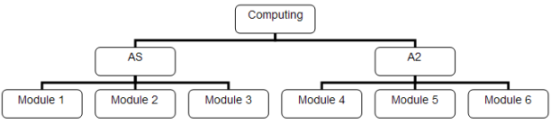# Computer ScienceTrees

## What Is A Tree?

A tree is a dynamic data structure in which data is stored in a hierarchical manner.In the above diagram, each part of the tree that contains information (the boxes) is called a node. The first node (in this diagram - 'Computing') is called the root node. The lines which connect each of the boxes are called branches. Some nodes have child nodes - they connect to nodes on the level below. Nodes that don't have children are called leaf nodes.

A tree is very similar to a graph. In fact, we could say that the tree shown above is a connected, undirected graph. This particular tree is a rooted tree. All of the edges are directed away from the root node.

## Binary Trees

A binary tree is a special type of tree where each node is allowed a maximum of 2 children. You construct a binary tree by placing the first data item as the root node. For each subsequent item, branch to the left if it is less than the root node, branch to the right if it is greater. Apply this branching rule at each node that is met unitl the item can be placed.

Do this with the following names,

Partridge, Duckworth, Smith, Arkwright, Wren, Thumbface, McJack.

You should end up with a tree diagram that looks like this,Starting from the root node, if you were searching for Thumbface, which items would you need to access?

Make another tree with the following captial cities,

London, Paris, Washington, Canberra, Lima, Madrid, Lisbon.

Which data items would be accessed if you were searching for Madrid?

## Traversing The Tree

There are a variety of methods used for traversing the tree to produce sorted output. The following traversal methods produce output in different orders.

• Pre-Order Traversal
• In-Order Traversal
• Post-Order Traversal

A diagrammatic representation of how to construct a binary search tree, as well as the different traversal methods, can be found by clicking or downloading the following PowerPoint slideshow. DOWNLOAD BINARY TREE SIMULATION

## Javascript Binary Tree Simulation

Remember that the diagrams shown in the slideshow are simply a way of representing the structure of the tree. In order to program with the tree, there is no need to replicate the visuals. In the example below an array of records is used. Each record has 3 properties, a name, a left pointer and a right pointer. The pointers indicate the address of the left or right child of a node. Add some names and watch how the pointers change as each item is added. Then press the buttons and see how they are sorted.

## Binary Tree Algorithms

The simulation on this page uses an array of records to manage the items in the binary tree. The structure definition is shown in C#, the remaining algorithms are pseudocode.

### Record Definition & Global Variables

```public struct TreeItem {    public int left;    public int right;    public string name; } int maxsize = 15; TreeItem[] treeitems = new TreeItem[maxsize];```

### Inserting Items In The Tree

Two procedures are used. The first checks that the tree is full and, if not then looks to see if this is the first item (root node) of the tree. If this is the root node it creates it, no fuss involved since the root node initially points to 0. If the node is not the root, the second procedure is called. This procedure is recursive, walking through the tree following the left/right rules changing the appropriate pointer of the parent node.

```Procedure InsertItem(itm)    IF treeitems.length = maxsize THEN Exit Procedure    IF treeitems.length = 0 THEN    treeitems = new TreeItem(itm)    ELSE       treeitems[treeitems.length] = new TreeItem(itm)       ChangePointers(treeitems.length - 1, 0)    END IF End Procedure``` ```Procedure ChangePointers(a,b)    IF treeitems[a].name < treeitems[b].name THEN       IF treeitems[b].left = 0 THEN          treeitems[b].left = a       ELSE          ChangePointers(a, treeitems[b].left)       END IF    ELSE       IF treeitems[b].right = 0 THEN          treeitems[b].right = a       ELSE          ChangePointers(a, treeitems[b].right)       END IF    END IF End Procedure```

### In-Order Traversal

The procedure for in-order traversal is recursive. The first call to this procedure would be InOrderPrint(0).

```Procedure InOrderPrint(a)    IF treeitems[a].left <> 0 THEN       InOrderPrint(treeitems[a].left)    END IF    Print treeitems[a].name    IF treeitems[a].right <> 0 THEN       InOrderPrint(treeitems[a].right)    END IF End Procedure```

### Pre-Order Traversal

```Procedure PreOrderPrint(a)    Print treeitems[a].name    IF treeitems[a].left <> 0 THEN       PreOrderPrint(treeitems[a].left)    END IF    IF treeitems[a].right <> 0 THEN       PreOrderPrint(treeitems[a].right)    END IF End Procedure```

### Post-Order Traversal

```Procedure PostOrderPrint(a)    IF treeitems[a].left <> 0 THEN       PostOrderPrint(treeitems[a].left)    END IF    IF treeitems[a].right <> 0 THEN       PostOrderPrint(treeitems[a].right)    END IF    Print treeitems[a].name End Procedure```